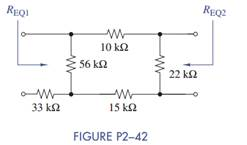### Create an Account

Already have account?

### Forgot Your Password ?

Home / Questions / Equivalent resistance is defined at a particular pair of terminal. In Figure P2 42 the sa...

# Equivalent resistance is defined at a particular pair of terminal. In Figure P2 42 the same circuit is looked at from two different terminal pairs Find the equivalent resistances

Equivalent resistance is defined at a particular pair of terminals. In Figure P2−42, the same circuit is looked at from two different terminal pairs. Find the equivalent resistances REQ1 and REQ2 in Figure P2−42. Note that in calculating REQ2 the 33-kΩ resistor is connected to an open circuit and therefore does not affect the calculation.Jun 15 2020 View more View Less

#### Answer (Solved)Subscribe To Get Solution